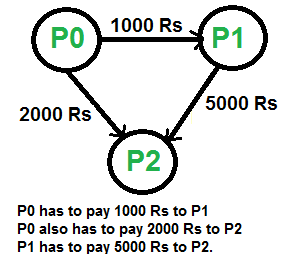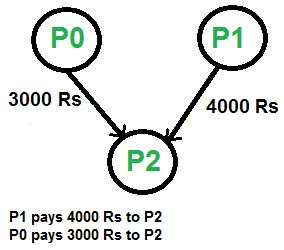# Minimize Cash Flow among a given set of friends who have borrowed money from each other

• Difficulty Level : Hard
• Last Updated : 04 Feb, 2022

Given a number of friends who have to give or take some amount of money from one another. Design an algorithm by which the total cash flow among all the friends is minimized.

Example:

Following diagram shows input debts to be settled.Above debts can be settled in following optimized wayThe idea is to use Greedy algorithm where at every step, settle all amounts of one person and recur for remaining n-1 persons.
How to pick the first person? To pick the first person, calculate the net amount for every person where net amount is obtained by subtracting all debts (amounts to pay) from all credits (amounts to be paid). Once net amount for every person is evaluated, find two persons with maximum and minimum net amounts. These two persons are the most creditors and debtors. The person with minimum of two is our first person to be settled and removed from list. Let the minimum of two amounts be x. We pay ‘x’ amount from the maximum debtor to maximum creditor and settle one person. If x is equal to the maximum debit, then maximum debtor is settled, else maximum creditor is settled.
The following is detailed algorithm.

Do following for every person Pi where i is from 0 to n-1.

1. Compute the net amount for every person. The net amount for person ‘i’ can be computed by subtracting sum of all debts from sum of all credits.
2. Find the two persons that are maximum creditor and maximum debtor. Let the maximum amount to be credited maximum creditor be maxCredit and maximum amount to be debited from maximum debtor be maxDebit. Let the maximum debtor be Pd and maximum creditor be Pc.
3. Find the minimum of maxDebit and maxCredit. Let minimum of two be x. Debit ‘x’ from Pd and credit this amount to Pc
4. If x is equal to maxCredit, then remove Pc from set of persons and recur for remaining (n-1) persons.
5. If x is equal to maxDebit, then remove Pd from set of persons and recur for remaining (n-1) persons.

Thanks to Balaji S for suggesting this method in a comment here.

The following is the implementation of the above algorithm.

## C++

 `// C++ program to find maximum cash flow among a set of persons``#include``using` `namespace` `std;` `// Number of persons (or vertices in the graph)``#define N 3` `// A utility function that returns index of minimum value in arr[]``int` `getMin(``int` `arr[])``{``    ``int` `minInd = 0;``    ``for` `(``int` `i=1; i arr[maxInd])``            ``maxInd = i;``    ``return` `maxInd;``}` `// A utility function to return minimum of 2 values``int` `minOf2(``int` `x, ``int` `y)``{``    ``return` `(x

## Java

 `// Java program to find maximum cash``// flow among a set of persons` `class` `GFG``{``    ``// Number of persons (or vertices in the graph)``    ``static` `final` `int` `N = ``3``;``    ` `    ``// A utility function that returns``    ``// index of minimum value in arr[]``    ``static` `int` `getMin(``int` `arr[])``    ``{``        ``int` `minInd = ``0``;``        ``for` `(``int` `i = ``1``; i < N; i++)``            ``if` `(arr[i] < arr[minInd])``                ``minInd = i;``        ``return` `minInd;``    ``}``    ` `    ``// A utility function that returns``    ``// index of maximum value in arr[]``    ``static` `int` `getMax(``int` `arr[])``    ``{``        ``int` `maxInd = ``0``;``        ``for` `(``int` `i = ``1``; i < N; i++)``            ``if` `(arr[i] > arr[maxInd])``                ``maxInd = i;``        ``return` `maxInd;``    ``}``    ` `    ``// A utility function to return minimum of 2 values``    ``static` `int` `minOf2(``int` `x, ``int` `y)``    ``{``        ``return` `(x < y) ? x: y;``    ``}``    ` `    ``// amount[p] indicates the net amount``    ``// to be credited/debited to/from person 'p'``    ``// If amount[p] is positive, then``    ``// i'th person will amount[i]``    ``// If amount[p] is negative, then``    ``// i'th person will give -amount[i]``    ``static` `void` `minCashFlowRec(``int` `amount[])``    ``{``        ``// Find the indexes of minimum and``        ``// maximum values in amount[]``        ``// amount[mxCredit] indicates the maximum amount``        ``// to be given (or credited) to any person .``        ``// And amount[mxDebit] indicates the maximum amount``        ``// to be taken(or debited) from any person.``        ``// So if there is a positive value in amount[],``        ``// then there must be a negative value``        ``int` `mxCredit = getMax(amount), mxDebit = getMin(amount);``    ` `        ``// If both amounts are 0, then``        ``// all amounts are settled``        ``if` `(amount[mxCredit] == ``0` `&& amount[mxDebit] == ``0``)``            ``return``;``    ` `        ``// Find the minimum of two amounts``        ``int` `min = minOf2(-amount[mxDebit], amount[mxCredit]);``        ``amount[mxCredit] -= min;``        ``amount[mxDebit] += min;``    ` `        ``// If minimum is the maximum amount to be``        ``System.out.println(``"Person "` `+ mxDebit + ``" pays "` `+ min``                                ``+ ``" to "` `+ ``"Person "` `+ mxCredit);``    ` `        ``// Recur for the amount array.``        ``// Note that it is guaranteed that``        ``// the recursion would terminate``        ``// as either amount[mxCredit]  or``        ``// amount[mxDebit] becomes 0``        ``minCashFlowRec(amount);``    ``}``    ` `    ``// Given a set of persons as graph[]``    ``// where graph[i][j] indicates``    ``// the amount that person i needs to``    ``// pay person j, this function``    ``// finds and prints the minimum``    ``// cash flow to settle all debts.``    ``static` `void` `minCashFlow(``int` `graph[][])``    ``{``        ``// Create an array amount[],``        ``// initialize all value in it as 0.``        ``int` `amount[]=``new` `int``[N];``    ` `        ``// Calculate the net amount to``        ``// be paid to person 'p', and``        ``// stores it in amount[p]. The``        ``// value of amount[p] can be``        ``// calculated by subtracting``        ``// debts of 'p' from credits of 'p'``        ``for` `(``int` `p = ``0``; p < N; p++)``        ``for` `(``int` `i = ``0``; i < N; i++)``            ``amount[p] += (graph[i][p] - graph[p][i]);``    ` `        ``minCashFlowRec(amount);``    ``}``    ` `    ``// Driver code``    ``public` `static` `void` `main (String[] args)``    ``{``        ``// graph[i][j] indicates the amount``        ``// that person i needs to pay person j``        ``int` `graph[][] = { {``0``, ``1000``, ``2000``},``                            ``{``0``, ``0``, ``5000``},``                            ``{``0``, ``0``, ``0``},};``    ` `        ``// Print the solution``        ``minCashFlow(graph);``    ``}``}` `// This code is contributed by Anant Agarwal.`

## Python3

 `# Python3 program to find maximum``# cash flow among a set of persons` `# Number of persons(or vertices in graph)``N ``=` `3` `# A utility function that returns``# index of minimum value in arr[]``def` `getMin(arr):``    ` `    ``minInd ``=` `0``    ``for` `i ``in` `range``(``1``, N):``        ``if` `(arr[i] < arr[minInd]):``            ``minInd ``=` `i``    ``return` `minInd` `# A utility function that returns``# index of maximum value in arr[]``def` `getMax(arr):` `    ``maxInd ``=` `0``    ``for` `i ``in` `range``(``1``, N):``        ``if` `(arr[i] > arr[maxInd]):``            ``maxInd ``=` `i``    ``return` `maxInd` `# A utility function to``# return minimum of 2 values``def` `minOf2(x, y):` `    ``return` `x ``if` `x < y ``else` `y` `# amount[p] indicates the net amount to``# be credited/debited to/from person 'p'``# If amount[p] is positive, then i'th``# person will amount[i]``# If amount[p] is negative, then i'th``# person will give -amount[i]``def` `minCashFlowRec(amount):` `    ``# Find the indexes of minimum``    ``# and maximum values in amount[]``    ``# amount[mxCredit] indicates the maximum``    ``# amount to be given(or credited) to any person.``    ``# And amount[mxDebit] indicates the maximum amount``    ``# to be taken (or debited) from any person.``    ``# So if there is a positive value in amount[],``    ``# then there must be a negative value``    ``mxCredit ``=` `getMax(amount)``    ``mxDebit ``=` `getMin(amount)` `    ``# If both amounts are 0,``    ``# then all amounts are settled``    ``if` `(amount[mxCredit] ``=``=` `0` `and` `amount[mxDebit] ``=``=` `0``):``        ``return` `0` `    ``# Find the minimum of two amounts``    ``min` `=` `minOf2(``-``amount[mxDebit], amount[mxCredit])``    ``amount[mxCredit] ``-``=``min``    ``amount[mxDebit] ``+``=` `min` `    ``# If minimum is the maximum amount to be``    ``print``(``"Person "` `, mxDebit , ``" pays "` `, ``min``        ``, ``" to "` `, ``"Person "` `, mxCredit)` `    ``# Recur for the amount array. Note that``    ``# it is guaranteed that the recursion``    ``# would terminate as either amount[mxCredit]``    ``# or amount[mxDebit] becomes 0``    ``minCashFlowRec(amount)` `# Given a set of persons as graph[] where``# graph[i][j] indicates the amount that``# person i needs to pay person j, this``# function finds and prints the minimum``# cash flow to settle all debts.``def` `minCashFlow(graph):` `    ``# Create an array amount[],``    ``# initialize all value in it as 0.``    ``amount ``=` `[``0` `for` `i ``in` `range``(N)]` `    ``# Calculate the net amount to be paid``    ``# to person 'p', and stores it in amount[p].``    ``# The value of amount[p] can be calculated by``    ``# subtracting debts of 'p' from credits of 'p'``    ``for` `p ``in` `range``(N):``        ``for` `i ``in` `range``(N):``            ``amount[p] ``+``=` `(graph[i][p] ``-` `graph[p][i])` `    ``minCashFlowRec(amount)``    ` `# Driver code` `# graph[i][j] indicates the amount``# that person i needs to pay person j``graph ``=` `[ [``0``, ``1000``, ``2000``],``          ``[``0``, ``0``, ``5000``],``          ``[``0``, ``0``, ``0``] ]` `minCashFlow(graph)` `# This code is contributed by Anant Agarwal.`

## C#

 `// C# program to find maximum cash``// flow among a set of persons``using` `System;` `class` `GFG``{``    ``// Number of persons (or``    ``// vertices in the graph)``    ``static` `int` `N = 3;``    ` `    ``// A utility function that returns``    ``// index of minimum value in arr[]``    ``static` `int` `getMin(``int` `[]arr)``    ``{``        ``int` `minInd = 0;``        ``for` `(``int` `i = 1; i < N; i++)``            ``if` `(arr[i] < arr[minInd])``                ``minInd = i;``        ``return` `minInd;``    ``}``    ` `    ``// A utility function that returns``    ``// index of maximum value in arr[]``    ``static` `int` `getMax(``int` `[]arr)``    ``{``        ``int` `maxInd = 0;``        ``for` `(``int` `i = 1; i < N; i++)``            ``if` `(arr[i] > arr[maxInd])``                ``maxInd = i;``        ``return` `maxInd;``    ``}``    ` `    ``// A utility function to return``    ``// minimum of 2 values``    ``static` `int` `minOf2(``int` `x, ``int` `y)``    ``{``        ``return` `(x < y) ? x: y;``    ``}``    ` `    ``// amount[p] indicates the net amount``    ``// to be credited/debited to/from person 'p'``    ``// If amount[p] is positive, then``    ``// i'th person will amount[i]``    ``// If amount[p] is negative, then``    ``// i'th person will give -amount[i]``    ``static` `void` `minCashFlowRec(``int` `[]amount)``    ``{``        ``// Find the indexes of minimum and``        ``// maximum values in amount[]``        ``// amount[mxCredit] indicates the maximum amount``        ``// to be given (or credited) to any person .``        ``// And amount[mxDebit] indicates the maximum amount``        ``// to be taken(or debited) from any person.``        ``// So if there is a positive value in amount[],``        ``// then there must be a negative value``        ``int` `mxCredit = getMax(amount), mxDebit = getMin(amount);``    ` `        ``// If both amounts are 0, then``        ``// all amounts are settled``        ``if` `(amount[mxCredit] == 0 &&``            ``amount[mxDebit] == 0)``            ``return``;``    ` `        ``// Find the minimum of two amounts``        ``int` `min = minOf2(-amount[mxDebit], amount[mxCredit]);``        ``amount[mxCredit] -= min;``        ``amount[mxDebit] += min;``    ` `        ``// If minimum is the maximum amount to be``        ``Console.WriteLine(``"Person "` `+ mxDebit +``                          ``" pays "` `+ min + ``" to "` `+``                          ``"Person "` `+ mxCredit);``    ` `        ``// Recur for the amount array.``        ``// Note that it is guaranteed that``        ``// the recursion would terminate``        ``// as either amount[mxCredit] or``        ``// amount[mxDebit] becomes 0``        ``minCashFlowRec(amount);``    ``}``    ` `    ``// Given a set of persons as graph[]``    ``// where graph[i][j] indicates``    ``// the amount that person i needs to``    ``// pay person j, this function``    ``// finds and prints the minimum``    ``// cash flow to settle all debts.``    ``static` `void` `minCashFlow(``int` `[,]graph)``    ``{``        ``// Create an array amount[],``        ``// initialize all value in it as 0.``        ``int` `[]amount=``new` `int``[N];``    ` `        ``// Calculate the net amount to``        ``// be paid to person 'p', and``        ``// stores it in amount[p]. The``        ``// value of amount[p] can be``        ``// calculated by subtracting``        ``// debts of 'p' from credits of 'p'``        ``for` `(``int` `p = 0; p < N; p++)``        ``for` `(``int` `i = 0; i < N; i++)``            ``amount[p] += (graph[i,p] - graph[p,i]);``    ` `        ``minCashFlowRec(amount);``    ``}``    ` `    ``// Driver code``    ``public` `static` `void` `Main ()``    ``{``        ``// graph[i][j] indicates the amount``        ``// that person i needs to pay person j``        ``int` `[,]graph = { {0, 1000, 2000},``                         ``{0, 0, 5000},``                         ``{0, 0, 0},};``    ` `        ``// Print the solution``        ``minCashFlow(graph);``    ``}``}` `// This code is contributed by nitin mittal.`

## PHP

 ` ``\$arr``[``\$maxInd``])``            ``\$maxInd` `= ``\$i``;``    ``return` `\$maxInd``;``}` `// A utility function to return minimum of 2 values``function` `minOf2(``\$x``, ``\$y``)``{``    ``return` `(``\$x` `< ``\$y``)? ``\$x``: ``\$y``;``}` `// amount[p] indicates the net amount``// to be credited/debited to/from person 'p'``// If amount[p] is positive, then i'th``// person will amount[i]``// If amount[p] is negative, then i'th``// person will give -amount[i]``function` `minCashFlowRec(``\$amount``)``{``    ``// Find the indexes of minimum and``    ``// maximum values in amount[]``    ``// amount[mxCredit] indicates the``    ``// maximum amount to be given``    ``// (or credited) to any person .``    ``// And amount[mxDebit] indicates the``    ``// maximum amount to be taken``    ``// (or debited) from any person.``    ``// So if there is a positive value in``    ``// amount[], then there must``    ``// be a negative value``    ``\$mxCredit` `= getMax(``\$amount``);``    ``\$mxDebit` `= getMin(``\$amount``);` `    ``// If both amounts are 0, then``    ``// all amounts are settled``    ``if` `(``\$amount``[``\$mxCredit``] == 0 &&``        ``\$amount``[``\$mxDebit``] == 0)``        ``return``;` `    ``// Find the minimum of two amounts``    ``\$min` `= minOf2(-``\$amount``[``\$mxDebit``], ``\$amount``[``\$mxCredit``]);``    ``\$amount``[``\$mxCredit``] -= ``\$min``;``    ``\$amount``[``\$mxDebit``] += ``\$min``;` `    ``// If minimum is the maximum amount to be``    ``echo` `"Person "``.``\$mxDebit``.``" pays "``.``\$min``.``" to Person "``.``\$mxCredit``.``"\n"``;` `    ``// Recur for the amount array. Note``    ``// that it is guaranteed that the``    ``// recursion would terminate as``    ``// either amount[mxCredit]``    ``// or amount[mxDebit] becomes 0``    ``minCashFlowRec(``\$amount``);``}` `// Given a set of persons as graph[]``// where graph[i][j] indicates the``// amount that person i needs to``// pay person j, this function finds``// and prints the minimum cash flow``// to settle all debts.``function` `minCashFlow(``\$graph``)``{``    ``global` `\$N``;``    ` `    ``// Create an array amount[],``    ``// initialize all value in it as 0.``    ``\$amount``=``array_fill``(0, ``\$N``, 0);` `    ``// Calculate the net amount to be``    ``// paid to person 'p', and stores``    ``// it in amount[p]. The value of``    ``// amount[p] can be calculated by``    ``// subtracting debts of 'p' from``    ``// credits of 'p'``    ``for` `(``\$p` `= 0; ``\$p` `< ``\$N``; ``\$p``++)``        ``for` `(``\$i` `= 0; ``\$i` `< ``\$N``; ``\$i``++)``            ``\$amount``[``\$p``] += (``\$graph``[``\$i``][``\$p``] - ``\$graph``[``\$p``][``\$i``]);` `    ``minCashFlowRec(``\$amount``);``}` `    ``// Driver code` `    ``// graph[i][j] indicates the amount``    ``// that person i needs to pay person j``    ``\$graph` `= ``array``(``array``(0, 1000, 2000),``                        ``array``(0, 0, 5000),``                        ``array``(0, 0, 0));` `    ``// Print the solution``    ``minCashFlow(``\$graph``);``    ` `// This code is contributed by mits``?>`

## Javascript

 ``

Output:

```Person 1 pays 4000 to Person 2
Person 0 pays 3000 to Person 2```